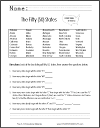﻿
Kindergarten: Operations and Algebraic Thinking | Student Handouts
 Share the learning joy!Kindergarten Math: Operations & Algebraic Thinking
Kindergarten > Kindergarten Mathematics > Kindergarten Operations and Algebraic Thinking

CCSS.MATH.CONTENT.K.OA.A.1 - Represent addition and subtraction with objects, fingers, mental images, drawings, sounds (e.g., claps), acting out situations, verbal explanations, expressions, or equations.

CCSS.MATH.CONTENT.K.OA.A.2 - Solve addition and subtraction word problems, and add and subtract within 10, e.g., by using objects or drawings to represent the problem.

CCSS.MATH.CONTENT.K.OA.A.3 - Decompose numbers less than or equal to 10 into pairs in more than one way, e.g., by using objects or drawings, and record each decomposition by a drawing or equation (e.g., 5 = 2 + 3 and 5 = 4 + 1).

CCSS.MATH.CONTENT.K.OA.A.4 - For any number from 1 to 9, find the number that makes 10 when added to the given number, e.g., by using objects or drawings, and record the answer with a drawing or equation.

CCSS.MATH.CONTENT.K.OA.A.5 - Fluently add and subtract within 5.50 States Math Worksheet